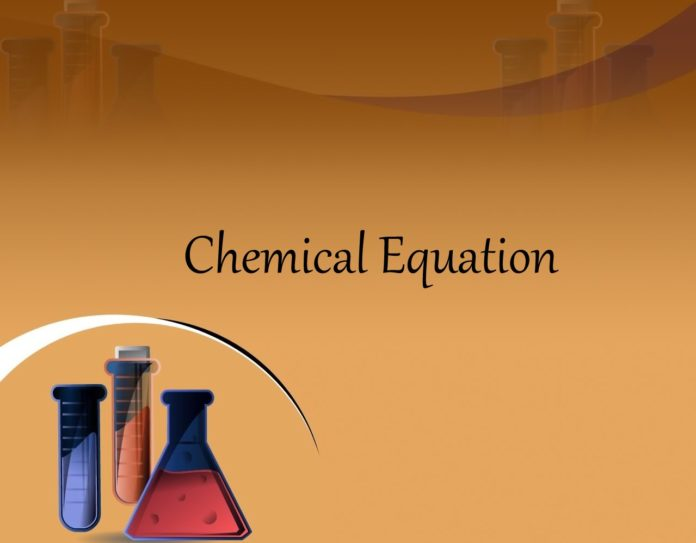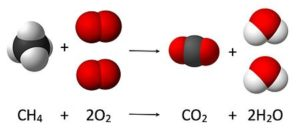What is a Chemical Equation:

A chemical equation is the symbolic representation of a chemical reaction in the form of symbols , where the reactants  are given on the left-hand side and the products  on the right-hand side. The coefficients next to the symbols of entities are the absolute values of the stoichiometric numbers.

2HCl + 2Na → 2NaCl + H2

This equation indicates that sodium and HCl react to produce NaCl and H2. It also indicates that two sodium molecules are required for every two hydrochloric acid molecules and the reaction will form two sodium chloride molecules and one molecule of hydrogen gas.

Reactants and Products:Reactant are the substances present at the beginning of a chemical reaction. In the burning of natural gas, for example, methane (CH4) and oxygen (O2) are the reactants in the chemical reaction.

Products are the substances formed by a chemical reaction. In the burning of natural gas, carbon dioxide (CO2) and water (H2O) are the products formed by the reaction.

Balanced Chemical Equation:

According to the law of conservation of mass, when a chemical reaction occurs, the mass of the products should be equal to the mass of the reactants. Therefore, the amount of the atoms in each element does not change in the chemical reaction. As a result, the chemical equation that shows the chemical reaction needs to be balanced. A balanced chemical equation occurs when the number of the atoms involved in the reactants side is equal to the number of atoms in the products side.

Steps to Balance an Equation

To balance an equation, here are the things we need to do:

• Count the atoms of each element in the reactants and the products.
• Use coefficients; place them in front of the compounds as needed.

Let’s take a example equation to balance:

HCl + Na → NaCl + H2

Reaction above is a chemical equation but not balanced. As amount molecules of elements are different in reactant and product.

As hydrogen(H) have molecule in product side. So multiply HCl by 2.

2HCl + Na → NaCl + H2

Now chlorine(Cl) have 2 molecules in reactant side. So multiply NaCl by 2 to balance Cl.

2HCl + Na → 2NaCl + H2

This time sodium(Na) have 2 molecules in product side. So multiply Na by 2.

2HCl + 2Na → 2NaCl + H2

This is a balanced chemical equation. As number of molecule in of each element is same both sides.